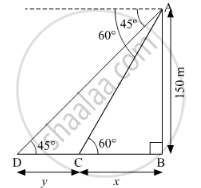Share

# A Moving Boat is Observed from the Top of a 150 M High Cliff Moving Away from the Cliff. the Angle of Depression of the Boat Changes from 60° to 45° in 2 Minutes. Find the Speed of the Boat in M/H - CBSE Class 10 - Mathematics

#### Question

A moving boat is observed from the top of a 150 m high cliff moving away from the cliff. The angle of depression of the boat changes from 60° to 45° in 2 minutes. Find the speed of the boat in m/h.

#### Solution

Let the distance BC be x m and CD be y m.In ΔABC

tan 60^@ = (AB)/(BC) = 150/x

=> sqrt3 = 150/x

=> x = 150/sqrt3 m ....(1)

In ΔABD

tan 45^@ = (AB)/(BD) = 150/(x + y)

=> 1 = 150/(x + y)

=> x + y = 150

=> y = 150 - x

Using 1 we get

=> y = 150 - 150/sqrt3 = (150(sqrt3 - 1))/sqrt3 m

Time taken to move  from the point C to point D is 2 min = 2/60 h = 1/30 h

Now.

Speed = "Distance"/"Time" = y/(1/30)

=(150((sqrt3-1))/sqrt3)/(1/30) = 1500 sqrt3 (sqrt3 - 1) "m/h"

Is there an error in this question or solution?

#### Video TutorialsVIEW ALL 

Solution A Moving Boat is Observed from the Top of a 150 M High Cliff Moving Away from the Cliff. the Angle of Depression of the Boat Changes from 60° to 45° in 2 Minutes. Find the Speed of the Boat in M/H Concept: Heights and Distances.
S